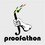# CMC - Problem 1

Problem 1 (5 points). Let $$a,b,c,d$$ be complex numbers satisfying

$a+b+c+d=42\text{,}$ $ab+ac+ad+bc+bd+cd=2013\text{, and}$ $a^3+b^3+c^3+d^3+abc+abd+acd+bcd=1337$

Find the last three digits of $a^4+b^4+c^4+d^4+4abcd$.Note by Cody Johnson
6 years, 8 months ago

This discussion board is a place to discuss our Daily Challenges and the math and science related to those challenges. Explanations are more than just a solution — they should explain the steps and thinking strategies that you used to obtain the solution. Comments should further the discussion of math and science.

When posting on Brilliant:

• Use the emojis to react to an explanation, whether you're congratulating a job well done , or just really confused .
• Ask specific questions about the challenge or the steps in somebody's explanation. Well-posed questions can add a lot to the discussion, but posting "I don't understand!" doesn't help anyone.
• Try to contribute something new to the discussion, whether it is an extension, generalization or other idea related to the challenge.

MarkdownAppears as
*italics* or _italics_ italics
**bold** or __bold__ bold
- bulleted- list
• bulleted
• list
1. numbered2. list
1. numbered
2. list
Note: you must add a full line of space before and after lists for them to show up correctly
paragraph 1paragraph 2

paragraph 1

paragraph 2

[example link](https://brilliant.org)example link
> This is a quote
This is a quote
    # I indented these lines
# 4 spaces, and now they show
# up as a code block.

print "hello world"
# I indented these lines
# 4 spaces, and now they show
# up as a code block.

print "hello world"
MathAppears as
Remember to wrap math in $$ ... $$ or $ ... $ to ensure proper formatting.
2 \times 3 $2 \times 3$
2^{34} $2^{34}$
a_{i-1} $a_{i-1}$
\frac{2}{3} $\frac{2}{3}$
\sqrt{2} $\sqrt{2}$
\sum_{i=1}^3 $\sum_{i=1}^3$
\sin \theta $\sin \theta$
\boxed{123} $\boxed{123}$

Sort by:

560

- 6 years, 8 months ago

Use Newton's Sum

We have $e_1 = p_1 = 42$

$2e_2 = e_1 p_1 - p_2 \Rightarrow 2(2013) = 42(42) - p_2 \Rightarrow p_2 = -2262$

$3e_3 = e_2 p_1 - e_1 p_2 + p_3 \Rightarrow 3e_3 = (2013)(42)-(42)(-2262) + p_3$

$\Rightarrow 3e_3 - p_3 = 179550$

Given $e_3 + p_3 = 1337$, solve them simultaneously gives $e_3 = 45221.75, p_3 = -43884.75$

Lastly,

4e_4 = e_3 p_1 - e_2 p_2 + e_1 p_3 - p_4

$4e_4 + p_4 = 45221.75(42) - 2013(-2262) + 42(-43884.75) = 4609560$

- 6 years, 8 months ago

Aaaaaand the 5 points goes to Pi Han Goh!

Official solution:

Let $a,b,c,d$ be roots of a polynomial

$f(x)=x^4-42x^3+2013x^2-(1337-(a^3+b^3+c^3+d^3))x+abcd)$

Add $f(a)+f(b)+f(c)+f(d)=0$ to get

\begin{aligned} 0&=a^4+b^4+c^4+d^4+4abcd-42(a^3+b^3+c^3+d^3)\\&+2013(42^2-2(2013))-1337(42)+42(a^3+b^3+c^3+d^3)\\&\equiv (a^4+b^4+c^4+d^4+4abcd)-560\pmod{1000} \end{aligned}

so that $a^4+b^4+c^4+d^4+4abcd\equiv\boxed{560}\pmod{1000}$

- 6 years, 8 months ago

Same solution; nice problem! Very similar to one of my Brilliant problems. ;)

- 6 years, 8 months ago

I think the answer is $\boxed{560}$

- 6 years, 8 months ago

That is correct! Solution?

- 6 years, 8 months ago

560

- 6 years, 8 months ago

- 6 years, 8 months ago

560

- 6 years, 8 months ago

I got....... a^{4} + b^{4} + c^{4} + d^{4} + 4abcd =4609560

Last digit are.....560

- 6 years, 8 months ago

a^{2} + b^{2} + c^{2} + d^{2} = -2262

- 6 years, 8 months ago

Post your answer only, then post a solution. Refer to the rules here.

- 6 years, 8 months ago

4(abc + abd + acd + bcd) = 180887

- 6 years, 8 months ago

3392

- 6 years, 8 months ago

2013+42+1337=3392

- 6 years, 8 months ago